New Features in Maple 17: ParallelismMaple 17 introduces parallelism into its memory management system, taking advantage of multiple processors to perform its job more quickly. This change can lead to a reduction of running times for all computations, not just parallel algorithms. With no code changes required, your computations will run 10% faster on average, with memory-intensive computations running up to 50% faster.

In addition, new programming constructs were added to make it easier to write parallel code.   Variables and procedure remember tables can be declared local to the thread, so that each instance can store different values. This allows for complex algorithms that need to maintain state to be written in a thread-safe manner.

Details and Examples

Parallel Memory Management

One of the core components of Maple's engine is the 'garbage collector'. The garbage collector is responsible for finding and reclaiming memory that is no longer needed by the evaluation engine. In Maple 17, the garbage collector is capable of taking advantage of multiple processors to perform its job more quickly. As the garbage collector is used continuously as Maple runs, this speed up helps all users, not just those running parallel algorithms.

• The garbage collector in Maple 17 is capable of running multiple threads to take advantage of multiple cores.  This can speed up the collector significantly.  Although the collector is only responsible for a fraction of Maple's total running time, this can still lead to running times reduced 10% on average, with memory-intensive computations running up to 50% faster.  These speed ups require no changes to user code.
• There are 2 kernelopts that can control the parallel collector.gcmaxthreads controls the maximum number of threads that the parallel garbage collector will use.gcthreadmemorysize controls how many threads Maple will use for a collection.  The number of bytes allocated is divided by the value assigned to gcthreadmemorysize to determine the number of threads.

Example

The following example shows the speed up in the garbage collector from using multiple threads.

 >>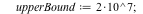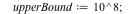>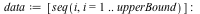>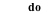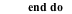>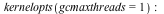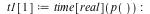>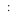>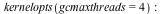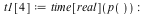>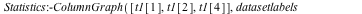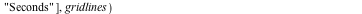Of course, the average compuation does not require such intensive memory management, so the garbage collection algorithm only comprises a small amount of the total Maple running time. But even in this more typical example, the improvement is significant.

 >>>>>>>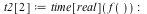>>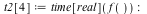>Thread Local Remember Tables

In Maple 17, procedures have different remember tables for each thread the procedure is executed on.  When a procedure with a thread local remember table is executed in parallel, modifications to the remember table will not lead to thread safety issues.  This change makes it easier to write thread safe functions that make use of remember tables, especially when direct manipulation of the remember table is required.

A procedure with a shared remember table, one that is shared between threads, can still be created by passing option shared to the procedure. This provides the same functionality as previous versions of Maple.

In Maple 17 remember tables are thread local by default.  When a procedure with a remember table is called by multiple threads, the remembered results are only available on the thread that created them.

The following procedures implement a sequence similar to Fibonacci, where each term is the sum of the previous two terms, however the user can specify the initial values.  A remember table is used to improve the performance of this procedure.  The initial values are stored in FibsHelper's remember table where they act as the termination condition for the recursion.

 > FibsHelper := proc (n::nonnegint) option remember; FibsHelper(n-1)+FibsHelper(n-2)  end proc;> Fibs := proc (n::nonnegint, t1 := 1, t2 := 1) subsop(4 = NULL, op(FibsHelper)); FibsHelper(0) := t1; FibsHelper(1) := t2; FibsHelper(n) end proc;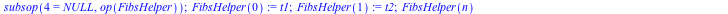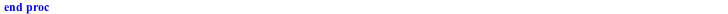> seq(Fibs(i), i = 0 .. 10);> seq(Fibs(i, 2, 2), i = 0 .. 10);> seq(Fibs(i, 2, 38), i = 0 .. 10);This works when run on a single thread, and in Maple 17, it works when run in parallel.

 > task := proc (n, t1, t2) local i; [seq(Fibs(i, t1, t2), i = 0 .. 10)] end proc;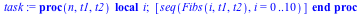> Threads:-Task:-Start(passed, Tasks = [task, [10, 1, 1], [10, 2, 2], [10, 2, 38]]);In previous versions of Maple, the parallel evaluation can fail.

A procedure with a shared remember table (Maple's previous behavior) can still be created in Maple 17 by specifying the shared option.  The following procedure will work when used in a single thread, but not in parallel.

 > SharedFibsHelper := proc (n::nonnegint) options remember, shared; SharedFibsHelper(n-1)+SharedFibsHelper(n-2) end proc;> SharedFibs := proc (n::nonnegint, t1 := 1, t2 := 1) subsop(4 = NULL, op(SharedFibsHelper)); SharedFibsHelper(0) := t1; SharedFibsHelper(1) := t2; SharedFibsHelper(n) end proc;> seq(SharedFibs(i), i = 0 .. 10);> seq(SharedFibs(i, 2, 2), i = 0 .. 10);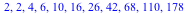> seq(SharedFibs(i, 2, 38), i = 0 .. 10);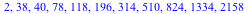When SharedFibs is called in parallel, incorrect results may be computed.  Note that due to the nature of parallel execution, incorrect results may not occur on every run.

 > sharedTask := proc (n, t1, t2) local i; [seq(SharedFibs(i, t1, t2), i = 0 .. 10)] end proc;> Threads:-Task:-Start(passed, Tasks = [sharedTask, [10, 1, 1], [10, 2, 2], [10, 2, 38]]);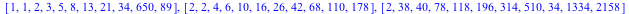There are various things that can go wrong.  Clearing the remember table in one thread can remove another thread's initial values, which will cause SharedFibsHelper to be called with invalid inputs (negative numbers).  One threads initial values can overwrite another threads, which will cause that thread to compute incorrect results.  One threads remembered values can be used by a different thread where those values are incorrect.

A procedure with a shared remember table can work correctly in parallel and computed results can be shared across threads.  However there are may complexities that require  procedures with shared remember tables to be written with care.  Maple 17's thread local remember tables makes it easier for procedure written for single threaded execution to work in parallel.

In Maple 17, module local variables can be declared thread local, meaning that they store a different value for each thread that access the variable. This allows for complex algorithms that need to maintain state to be written in a thread safe manner.

A module that wants to maintain internal state generally will not work correctly when executed in parallel. Consider the following example: the Mapper module maintains an internal state using the variables func and data.  The setMapper routine is used to set these variables. When ModuleApply is called, it maps func over the given data.

 > Mapper := module () local func, data; export setMapper, ModuleApply;  setMapper := proc (f::procedure, d::anything) func := f; data := d; NULL end proc; ModuleApply := proc (d) map(func, d, data) end proc end module:

 > adder := proc () Mapper:-setMapper(proc (x, y) options operator, arrow; x+y end proc, 1); Mapper([seq(i, i = 1 .. 10)]) end proc:> multer := proc () Mapper:-setMapper(proc (x, y) options operator, arrow; x*y end proc, 3); Mapper([seq(i, i = 1 .. 10)]) end proc:

 > multer();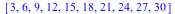If you execute the adder and multer commands in parallel, you can get incorrect results. Executing the following statement multiple times will show different results, some of which will be incorrect.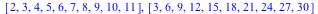Incorrect results are computed because the func and data state variables are shared between threads. Thus when one thread changes func's value, the other thread is also affected. In Maple 17, this can be fixed by declaring the state variables as thread_local. This means that each thread will maintain its own value for the state variables. Thus changing the value in one thread will not effect other threads.

 > MapperTL := module () local func::thread_local, data::thread_local; export setMapper, ModuleApply;   setMapper := proc (f::procedure, d::anything) func := f; data := d; NULL end proc; ModuleApply := proc (d) map(func, d, data) end proc end module;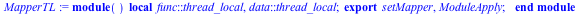> adderTL := proc () MapperTL:-setMapper(proc (x, y) options operator, arrow; x+y end proc, 1); MapperTL([seq(i, i = 1 .. 10)]) end proc;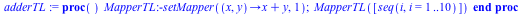> multerTL := proc () MapperTL:-setMapper(proc (x, y) options operator, arrow; x*y end proc, 3); MapperTL([seq(i, i = 1 .. 10)]) end proc;> multerTL();Executing this version, using the thread local variables, will return the expected result.